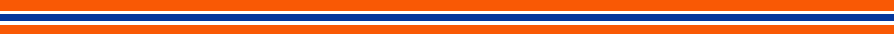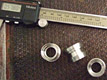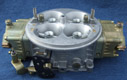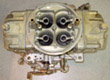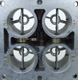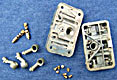Analyzing Dyno Data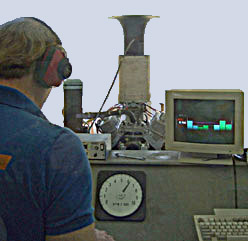Many times I have been asked to make sense out of incomplete or sketchy dyno data. Some engine builders use the data acquisition system on their dynos to “enhance” the horsepower numbers of their engines. Some of their customers suspect that something is wrong with the numbers when their engines don’t perform to the expectations they have, based on those numbers.

In some cases there is enough data provided to “calculate backwards” to determine the actual power of the engine. If a dyno sheet has data for fuel flow and brake specific fuel consumption (BSFC) for each RPM where data is taken, then the actual or “observed horsepower” (uncorrected for atmospheric conditions or any thing else) can be calculated. After all, it is “observed power” that moves the racing vehicle on the track, not corrected power. Let’s take, for example, a dyno data sheet that shows that an engine, at peak horsepower (let‘s say 7000 RPM), is using 301.5 #/hr (pounds per hour) and has a BSFC of .45. To calculate the actual power that the engine made at that RPM, divide the fuel flow by the BSFC.

301.5/.45= 670 HP

Now, that is the actual horsepower made at that RPM with the atmospheric conditions that existed at the time of that dyno run. Some dyno data sheets will show the atmospheric conditions, some don’t. If that data is not shown on the sheet, that is our first clue that the dyno operator is trying to hide something. Data on atmospheric conditions (“weather data”) should include actual atmospheric pressure, data on % humidity or vapor pressure, and actual temperature at the time of the run. For the effect of humidity on the atmospheric correction factor, I use the vapor pressure of the water in the air. This is expressed in inches of mercury, as is the barometric pressure. I calculate the correction factor (C.F.) with this equation.

Barometric Pressure=BP
Vapor Pressure=VP                                    C.F.=   29.92    x   (Temp F + 459)
Temperature= Temp Fahrenheit                    (BP -VP)                519

This correction factor corrects the power numbers to what the engine would make at sea level on a normal weather day. The S.A.E. standard calls for normal sea level weather to have a barometric pressure of 29.92”Hg, a temperature of 60 F, and 0% humidity. If the dyno sheet does not show weather data, the correction factor can be estimated if the location of the engine testing is known. Once a reasonable C.F. is determined, then the corrected horsepower is calculated by multiplying the observed HP by the C.F. . Here in Tucson, the average C.F. in the spring is about 1.12. So if we use this C.F., our example engine that makes 670 observed HP, makes 670 x 1.12 = 750.4 corrected HP .

When a dyno has a data acquisition system, most of the testing is short acceleration runs, usually less than 15 seconds of full throttle testing per run. It’s not likely that the weather data is changing during that short period of time for one run. So the C.F. that is calculated applies to all of the observed HP numbers that are recorded during the run. If you find that the corrected HP numbers on a dyno sheet get progressively further over what you are calculating as the RPM goes up, then the dyno operator had some other factors at work to enhance the numbers. The most common of these are the inertia factor and the friction factor. Inertia factor, when it is properly set, is a legitimate thing. But it can be set to artificially enhance the numbers. Friction factor, in my way of thinking, is somewhat phony.To be legitimate, the internal friction of the engine must be well known. Most often, this internal friction is just a rough estimate. The friction factor can be manipulated to grossly inflate the corrected power numbers. The misuse of these factors is a dishonest practice. You can come to your own conclusions about why some dyno operators use them this way.

I hope this gives you some capability in analyzing dyno sheets you may get to see. This may give you some insight into who is honest and who isn’t. It may surprise you.